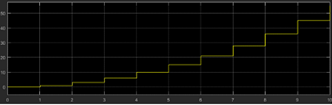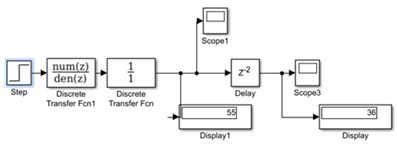# Welcome to the Digital Signal Processing MCQs Page

Dive deep into the fascinating world of Digital Signal Processing with our comprehensive set of Multiple-Choice Questions (MCQs). This page is dedicated to exploring the fundamental concepts and intricacies of Digital Signal Processing, a crucial aspect of MATLAB. In this section, you will encounter a diverse range of MCQs that cover various aspects of Digital Signal Processing, from the basic principles to advanced topics. Each question is thoughtfully crafted to challenge your knowledge and deepen your understanding of this critical subcategory within MATLAB.

Check out the MCQs below to embark on an enriching journey through Digital Signal Processing. Test your knowledge, expand your horizons, and solidify your grasp on this vital area of MATLAB.

Note: Each MCQ comes with multiple answer choices. Select the most appropriate option and test your understanding of Digital Signal Processing. You can click on an option to test your knowledge before viewing the solution for a MCQ. Happy learning!

### Digital Signal Processing MCQs | Page 1 of 8

Q1.
What is the following graph if u(t) denotes step function?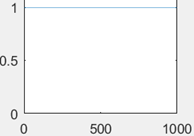Q2.
What is the following graph if u(t) denotes step function and r(t) denotes ramp function?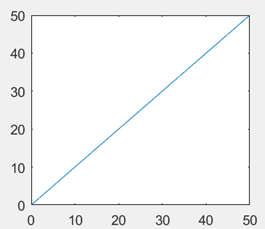Q3.
What is the following graph, if del(t) represents the impulse function?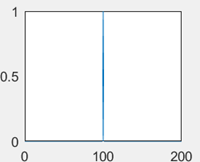Q4.
What is the Scope value if the signal generator has a frequency of 2 Hz only?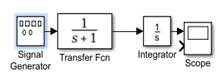Answer: (a).An attenuated signal of same frequency
Q5.
What will be seen in the scope if the step time is 1 second in the following Simulink Model?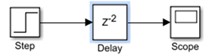Q6.
What will be seen in the scope if the step time is 0 seconds in the following Simulink Model?Answer: (a).A unit step signal delayed by 2 units in time
Q7.
What will be seen in the Scope if the step time is 0 seconds in the following Simulink Model and the limit of x axis is n=10?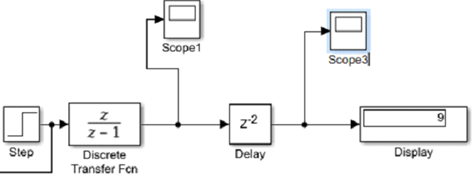Q8.
How much does the delay change, if the step time is taken as two units in time, in r(t-m), in scope 1 and 3?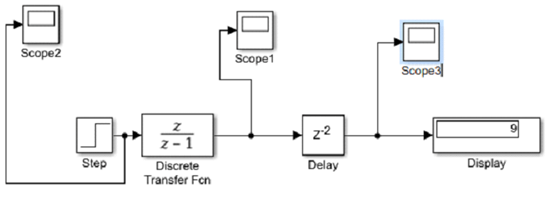Q9.
This is the output of scope1. What is the transfer function1?